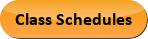Aug 14, 2022
2022-2023 College Catalog
 Select a Catalog 2022-2023 College Catalog 2021-2022 College Catalog [ARCHIVED CATALOG] 2020-2021 College Catalog [ARCHIVED CATALOG] 2019-2020 College Catalog [ARCHIVED CATALOG]
 HELP 2022-2023 College Catalog Print-Friendly Page (opens a new window)

# ENG 260 - Electrical Engineering

3 Credits, 3 Contact Hours
3 lecture periods 0 lab periods

Introductory survey of the electrical engineering discipline with emphasis on electrical power applications. Includes resistive circuits, inductance and capacitance, transients, steady-state sinusoidal analysis, and logic circuits. Also includes operational amplifiers, microcomputers, and diode electronics.

Prerequisite(s): MAT 231  and PHY 216IN .Course Learning Outcomes
1. Demonstrate the ability to apply Node Voltage Analysis and Mesh Current Analysis equations for circuits containing dependent and independent sources and resistors.
2. Demonstrate the ability to reduce complex circuits to Thevenin (or Norton) equivalent circuits.
3. Demonstrate the ability to use transient analysis to find the value of any current or voltage in resistor-inductor (RL), resistor-capacitor (RC), and resistor-inductor-capacitor (RCL) circuits.
4. Demonstrate the ability to apply phasors to solve mesh and node problems in alternating current (AC) circuits.
5. For a given combination of load impedance, applied voltage and through-current, demonstrate the ability to determine any of the following quantities: average and reactive power, power factor, complex power, and rms voltage and current values.

Performance Objectives:
1. Apply the passive sign convention to calculate power in an ideal circuit element and state whether the power is being absorbed or delivered.
2. Apply Kirchoff’s voltage law and current law (KVL/KCL) to simple circuits.
3. Apply parallel and series relationships to find the equivalent resistance of complex resistor networks and the equivalent source when sources are corrected in series and parallel.
4. Apply KVL/KCL to solve single node/mesh (loop) circuit problems, such as finding an unknown voltage, current or power.
5. Write and solve Node Voltage Analysis and Mesh Current Analysis equations for circuits containing dependent and independent sources and resistors.
6. Describe opportunities for applying source transformations and explain why source transformations are useful in circuit analysis.
7. Reduce complex circuits to Thevenin (or Norton) equivalent circuits, and explain the physical significance of the internal resistance and voltage (or current) quantities.
8. Apply v-i formulae for inductors and capacitors to find voltage when current is specified, and vice-versa; and find the equivalent component value when multiple capacitors and inductors are connected in series/parallel.
9. Explain in both qualitative and quantitative terms why the state variable in an inductor or capacitor resists abrupt change.
10. Use the formula sheet to find the value of any current or voltage in resistor inductor (RL) and resistance capacitor (RC) circuits.
11. Given a parallel or series resonant circuit (RLC) circuit, the circuit’s initial conditions, and a step excitation, find the node voltage (parallel RLC) or loop current (series RLC).
12. Write any given sinusoid as a phasor, and vice-versa and draw phasor diagrams for circuits with resistor (R), inductor (L), and capacitor (C) components.
13. Apply phasors to find Thevenin/Norton equivalents and solve mesh and node problems in alternating current (ac) circuits.
14. Given a combination of load impedance, applied voltage and through-current, find any of these quantities: average and reactive power, power factor, complex power, and rms voltage and current values.
15. Analyze three-phase circuits in Y-Y connection.
16. List the essential terminal characteristics of an ideal op-amp, and apply these to calculate voltage and current quantities in op-amp circuits with and without feedback resistance connected.
17. Describe a design problem in digital logic form and use a truth table to verify a Boolean expression.
18. Using a truth table, write a Boolean expression in sums of products (SOP) and product of sums (POS) form and construct a digital circuit using AND and OR gates.
19. Explain about computer architecture and essential computer subsystems.
20. List the type of microprocessors and memory types used in various electronic devices.
21. Explain the role of microcomputers in control systems.
22. Describe methods to program microprocessors for various operations.
23. Identify the basics of diode in electronic instrumentations.
24. Explain the characteristic of various diode.
25. Analyze a rectifier circuit.
26. Discuss small signal analysis of diode and its applications

Outline:
1. Introduction
1. Overview of electrical engineering
2. Circuits, currents, and voltages
3. Power and energy
4. Kirchhoff’s current law
5. Kirchhoff’s voltage law
6. Introduction to circuit elements
7. Introduction to circuits
2. Resistive Circuits
1. Resistances in series and parallel
2. Network analysis by using series and parallel equivalents
3. Voltage-divider and current-divider circuits
4. Node-voltage analysis
5. Mesh-current analysis
6. Thevenin and Norton equivalent circuits
7. Superposition principle
3. Inductance and Capacitance
1. Capacitances in series and parallel
2. Physical characteristics of capacitors
3. Inductance
4. Inductances in series and parallel
5. Practical inductors
6. Mutual inductance
4. Transients
1. Direct current (DC) steady state
2. RL circuits
3. RC and RL circuits with general sources
4. RLC second-order circuits
1. Phasors
2. Complex impedances
3. Circuit analysis with phasors and complex impedances
4. Power in alternating current (AC) circuits
5. Thevenin and Norton equivalent circuits
6. Balanced three-phase circuits
6. Logic Circuits
1. Basic logic circuit concepts
2. Representation of numerical data in binary form
3. Combinatorial logic circuits
4. Synthesis of logic circuits
7. Operational Amplifiers
1. Ideal operational amplifiers
2. Summing-point constraint
3. Inverting amplifiers
4. Noninverting amplifiers
5. Design of simple amplifiers
8. Microcomputers
1. Microcomputer organization
2. Microprocessor types
3. Memory types
4. Digital process control
5. Machine code and assembly languages
9. Diode Electronics
1. Diode concepts and operations
2. Diode types and load line characteristics
3. Ideal and piecewise-linear diode model
4. Rectifier circuits

Effective Term: